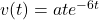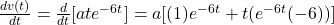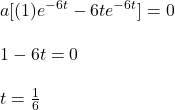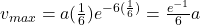## The instantaneous speed of a particle moving along one straight line is v(t) = ate−6t, where the speed v is measured in meters per second, t

Question

The instantaneous speed of a particle moving along one straight line is v(t) = ate−6t, where the speed v is measured in meters per second, the time t is measured in seconds, and the magnitude of the constant a is measured in meters per second squared. What is its maximum speed, expressed as a multiple of a? (Do not include units in your answer.)

in progress 0
4 months 2021-09-05T09:40:49+00:00 1 Answers 2 views 0

v_max = (1/6)e^-1 a

Explanation:

You have the following equation for the instantaneous speed of a particle:(1)

To find the expression for the maximum speed in terms of the acceleration “a”, you first derivative v(t) respect to time t:(2)

where you have use the derivative of a product.

Next, you equal the expression (2) to zero in order to calculate t:For t = 1/6 you obtain the maximum speed.

Then, you replace that value of t in the expression (1):hence, the maximum speed is v_max = ((1/6)e^-1)a